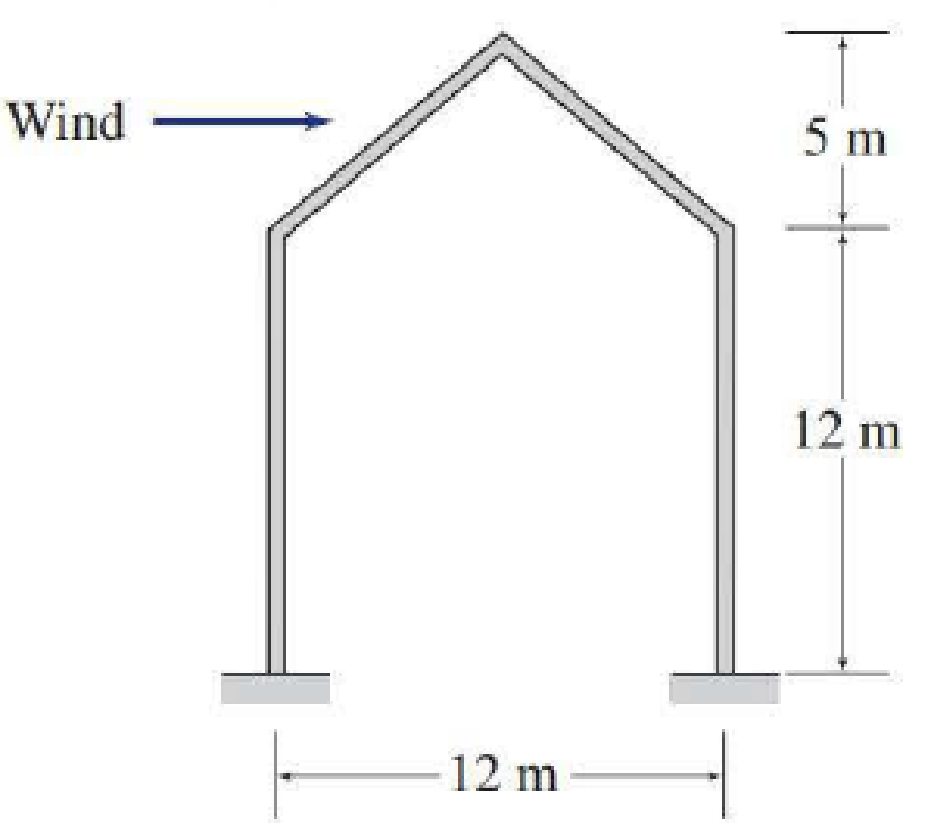# Determine the external wind pressure on the roof of the rigid-gabled frame of a school building shown in Fig. P2.13. The structure is located in a suburb of Chicago, Illinois, where the terrain is representative of exposure B and the basic wind speed for risk category III buildings is 51 m/ s. Assume the wind direction is normal to the ridge as shown. Use K d = 0.85 for main wind-force resisting systems, and K e = 1. FIG. P2.13, P2.17

#### Solutions

Chapter
Section
Chapter 2, Problem 13P
Textbook Problem
107 views

## Determine the external wind pressure on the roof of the rigid-gabled frame of a school building shown in Fig. P2.13. The structure is located in a suburb of Chicago, Illinois, where the terrain is representative of exposure B and the basic wind speed for risk category III buildings is 51 m/ s. Assume the wind direction is normal to the ridge as shown. Use Kd = 0.85 for main wind-force resisting systems, and Ke = 1.FIG. P2.13, P2.17

To determine

Find the external wind pressure acting on the windward and leeward side of the roof of the building.

### Explanation of Solution

Given information:

The terrain of the city belongs to Exposure B.

The building belongs to risk category III.

The basic wind speed is V=51m/s.

The direction of the wind is normal to the ridge.

Calculation:

Show the plan and elevation of the building as shown in Figure 1.

Refer Figure 1.

Calculate the angle of slope (θ) of the roof using the relation:

tanθ=5m6mθ=tan1(0.833)θ=39.8°

Calculate the mean roof height (h) as follows:

h=12m+5m2=14.5m

Calculate the value of (hL) as follows:

Substitute 14.5m for h and 12m for L.

hL=14.512=1.20831.21

Refer the Table 2.4 “Exposure Categories for Buildings for Wind Loads” in the textbook.

For exposure B.

The values of gradient height is zg=365.76m.

The value of power law coefficient is α=7.

Show the expression for the velocity pressure coefficient (Kh) as follows:

Kh=2.01(hzg)2α (1)

Substitute 14.5m for h, 365.76m for zg, and 7 for α in Equation (1).

Kh=2.01(14.5365.76)27=2.01×0.39762=0.79920.8

The value of the topographic factor (Kzt) as 1 for main wind force resisting system.

The value of the wind directionality factor (Kd) is 0.85 for main wind force resisting system.

The value of the ground elevation factor (Ke) is 1 for ground elevation at sea level.

Show the expression for the velocity pressure (qh) at height h as follows:

qh=0.613KhKztKdKeV2 (2)

Here, qh is in N/m2.

Substitute 0.8 for Kh, 1 for Kzt, 0.85 for Kd, 1 for Ke, and 51m/s for V in Equation (2).

qh=0.613×0.8×1×0.85×1×512=1084.2N/m2

Take the value of the gust effect factor (G) as 0.85 for rigid structures.

Consider windward Side of the roof.

Refer Figure 2

### Still sussing out bartleby?

Check out a sample textbook solution.

See a sample solution

#### The Solution to Your Study Problems

Bartleby provides explanations to thousands of textbook problems written by our experts, many with advanced degrees!

Get Started

Find more solutions based on key concepts
What are interesttime factors, and how are they used in the analysis of engineering economics problems?

Engineering Fundamentals: An Introduction to Engineering (MindTap Course List)

Explain how to use a push stick.

Precision Machining Technology (MindTap Course List)

Define an actor, and provide three examples.

Systems Analysis and Design (Shelly Cashman Series) (MindTap Course List)

Why might users work with a sandbox? (525)

Enhanced Discovering Computers 2017 (Shelly Cashman Series) (MindTap Course List)

If your motherboard supports ECC DDR3 memory, can you substitute non-ECC DDR3 memory?

A+ Guide to Hardware (Standalone Book) (MindTap Course List)